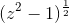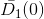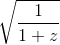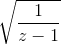Home / Answered Questions / Other / consider-the-multivalued-function-f-z-b-define-a-branch-of-f-that-is-holomorphic-on-the-set-c-z-c-z--aw772

# (Solved): Consider The Multivalued Function F(z) = . (b) Define A Branch Of F That Is Holomorphic On The Set C...

Consider the multivalued function f(z) =.

(b) Define a branch of f that is holomorphic on the set C= {z âˆˆC : |z| > 1}.

the hint tell me observe that z^2âˆ’1 = z^2(1âˆ’z^(âˆ’2)) for non-zero z, I personal think it should beand.

(2 - 1) D(0) V1+2 V 2 - 1

We have an Answer from Expert